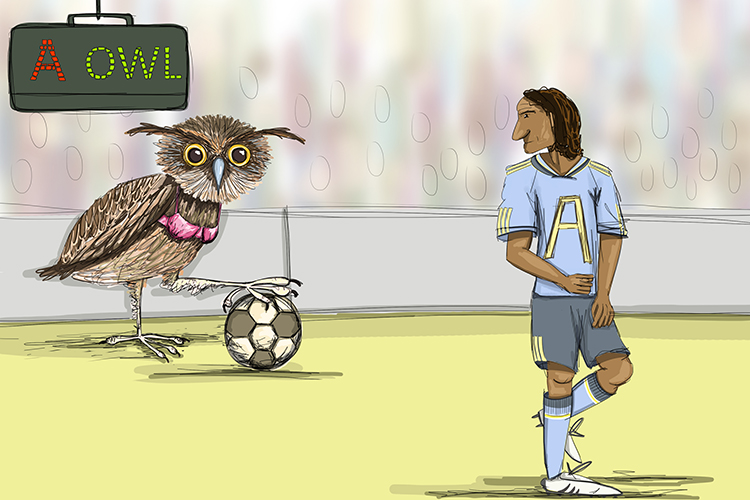# Algebra

Algebra – Substitutes letters for numbers.

It deals with symbols and the rules.The owl with a bra (algebra) was the substitute for one of the footballers with a letter on their shirt.

Example 1

One of the most famous algebraic expressions is:

E=mc^2

The letters E (energy) and m (mass) are variables and the letter c is the speed of light which is a constant.

Example 2

ax^2+bx+c=0

A famous algebraic equation is the quadratic equation.

## Remember Algebra

Another way to help you remember that algebra is substituting letters for numbers is as follows:Along with being a footballer she’s always having problems in the papers with her eX’s (X).